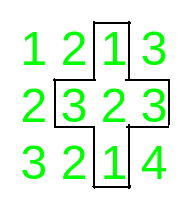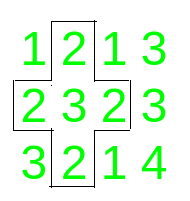# Count of palindromic plus paths in a given Matrix

Given an N x M matrix of integers, the task is to count the number of palindromic pluses in the array.

Palindromic plus is formed when a palindromic sub-row and palindromic sub-column cross each other at the middle element.

Examples:

Input: matrix = [[1, 2, 1], [2, 3, 2], [3, 2, 1]]
Output: 1
Explanation:
Palindromic row from (1, 0) – > (1, 2) and Palindromic column (0, 1) -> (2, 1) form a palindromic plus.

Input: matrix = [[1, 2, 1, 3], [2, 3, 2, 3], [3, 2, 1, 4]
Output: 2
Explanation:
The palindromic pluses in the given matrix are:## Recommended: Please try your approach on {IDE} first, before moving on to the solution.

Approach:
To solve the problem, follow the steps below:

• Traverse all the cells that can be the center of a palindromic plus, that is, all the cells apart from the ones belonging to the first and last row and columns.
• For all these cells (i, j), check if a[i][j – 1] is equal to a[i][j + 1] and a[i – 1][j] is equal to a[i + 1][j]. If both the conditions satisfies, then increase the count of palindromic pluses.
• Print the final count of palindromic pluses.

Below is the implementation of the above approach:

## C++

 `// C++ Program to count the number ` `// of palindromic pluses in ` `// a given matrix ` `#include ` `using` `namespace` `std; ` ` `  `// Function to count and return ` `// the number of palindromic pluses ` `int` `countPalindromicPlus( ` `    ``int` `n, ``int` `m, ` `    ``vector >& a) ` `{ ` `    ``int` `i, j, k; ` `    ``int` `count = 0; ` ` `  `    ``// Traverse all the centers ` `    ``for` `(i = 1; i < n - 1; i++) { ` `        ``for` `(j = 1; j < m - 1; j++) { ` ` `  `            ``// Check for palindromic plus ` `            ``// Check whether row and ` `            ``// column are palindrome or not ` `            ``if` `(a[i + 1][j] == a[i - 1][j] ` `                ``&& a[i][j - 1] == a[i][j + 1]) ` `                ``++count; ` `        ``} ` `    ``} ` `    ``// Return the answer ` `    ``return` `count; ` `} ` ` `  `// Driver code ` `int` `main() ` `{ ` `    ``int` `n = 4, m = 4; ` ` `  `    ``vector > a ` `        ``= { { 1, 2, 1, 3 }, ` `            ``{ 2, 3, 2, 3 }, ` `            ``{ 3, 2, 1, 2 }, ` `            ``{ 2, 3, 2, 3 } }; ` `    ``cout << countPalindromicPlus( ` `                ``n, m, a) ` `         ``<< endl; ` ` `  `    ``return` `0; ` `} `

## Java

 `// Java program to count the number ` `// of palindromic pluses in ` `// a given matrix ` `class` `GFG{ ` ` `  `// Function to count and return ` `// the number of palindromic pluses ` `static` `int` `countPalindromicPlus(``int` `n, ``int` `m, ` `                                ``int` `[][]a) ` `{ ` `    ``int` `i, j; ` `    ``int` `count = ``0``; ` ` `  `    ``// Traverse all the centers ` `    ``for``(i = ``1``; i < n - ``1``; i++) ` `    ``{ ` `       ``for``(j = ``1``; j < m - ``1``; j++) ` `       ``{ ` `           `  `          ``// Check for palindromic plus ` `          ``// Check whether row and ` `          ``// column are palindrome or not ` `          ``if` `(a[i + ``1``][j] == a[i - ``1``][j] &&  ` `              ``a[i][j - ``1``] == a[i][j + ``1``]) ` `              ``++count; ` `       ``} ` `    ``} ` `     `  `    ``// Return the answer ` `    ``return` `count; ` `} ` ` `  `// Driver code ` `public` `static` `void` `main(String[] args) ` `{ ` `    ``int` `n = ``4``, m = ``4``; ` `    ``int` `[][]a = { { ``1``, ``2``, ``1``, ``3` `}, ` `                  ``{ ``2``, ``3``, ``2``, ``3` `}, ` `                  ``{ ``3``, ``2``, ``1``, ``2` `}, ` `                  ``{ ``2``, ``3``, ``2``, ``3` `} }; ` `                   `  `    ``System.out.print( ` `           ``countPalindromicPlus(n, m, a) + ``"\n"``); ` `} ` `} ` ` `  `// This code is contributed by amal kumar choubey `

## Python3

 `# Python3 Program to count the number ` `# of palindromic pluses in ` `# a given matrix ` ` `  `# Function to count and return ` `# the number of palindromic pluses ` `def` `countPalindromicPlus(n, m, a): ` `    ``i, j, k ``=` `0``, ``0``, ``0` `    ``count ``=` `0` ` `  `    ``# Traverse all the centers ` `    ``for` `i ``in` `range``(``1``, n ``-` `1``): ` `        ``for` `j ``in` `range``(``1``, m ``-` `1``): ` ` `  `            ``# Check for palindromic plus ` `            ``# Check whether row and ` `            ``# column are palindrome or not ` `            ``if` `(a[i ``+` `1``][j] ``=``=` `a[i ``-` `1``][j] ` `                ``and` `a[i][j ``-` `1``] ``=``=` `a[i][j ``+` `1``]): ` `                ``count ``+``=` `1` `                 `  `    ``# Return the answer ` `    ``return` `count ` ` `  `# Driver code ` `if` `__name__ ``=``=` `'__main__'``: ` `    ``n ``=` `4` `    ``m ``=` `4` ` `  `    ``a ``=` `[[``1``, ``2``, ``1``, ``3` `], ` `         ``[``2``, ``3``, ``2``, ``3` `], ` `         ``[``3``, ``2``, ``1``, ``2` `], ` `         ``[``2``, ``3``, ``2``, ``3` `]] ` `    ``print``(countPalindromicPlus(n, m, a)) ` ` `  `# This code is contributed by Mohit Kumar `

## C#

 `// C# program to count the number ` `// of palindromic pluses in ` `// a given matrix ` `using` `System; ` `class` `GFG{ ` ` `  `// Function to count and return ` `// the number of palindromic pluses ` `static` `int` `countPalindromicPlus(``int` `n, ``int` `m, ` `                                ``int` `[,]a) ` `{ ` `    ``int` `i, j; ` `    ``int` `count = 0; ` ` `  `    ``// Traverse all the centers ` `    ``for``(i = 1; i < n - 1; i++) ` `    ``{ ` `        ``for``(j = 1; j < m - 1; j++) ` `        ``{ ` `             `  `            ``// Check for palindromic plus ` `            ``// Check whether row and ` `            ``// column are palindrome or not ` `            ``if` `(a[i + 1, j] == a[i - 1, j] &&  ` `                ``a[i, j - 1] == a[i, j + 1]) ` `                ``++count; ` `        ``} ` `    ``} ` `     `  `    ``// Return the answer ` `    ``return` `count; ` `} ` ` `  `// Driver code ` `public` `static` `void` `Main() ` `{ ` `    ``int` `n = 4, m = 4; ` `    ``int` `[,]a = {{ 1, 2, 1, 3 }, ` `                ``{ 2, 3, 2, 3 }, ` `                ``{ 3, 2, 1, 2 }, ` `                ``{ 2, 3, 2, 3 }}; ` `                 `  `    ``Console.Write( ` `            ``countPalindromicPlus(n, m, a) + ``"\n"``); ` `} ` `} ` ` `  `// This code is contributed by Code_Mech `

Output:

```3
```

Time Complexity: O(N2)
Auxiliary Space: O(1)My Personal Notes arrow_drop_upCheck out this Author's contributed articles.

If you like GeeksforGeeks and would like to contribute, you can also write an article using contribute.geeksforgeeks.org or mail your article to contribute@geeksforgeeks.org. See your article appearing on the GeeksforGeeks main page and help other Geeks.

Please Improve this article if you find anything incorrect by clicking on the "Improve Article" button below.